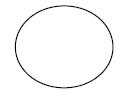# CBSE Class 5 Mathematics Geometric Constructions MCQs

## Geometric Constructions Class 5 Mathematics MCQ

Class 5 Mathematics students should refer to the following multiple-choice questions with answers for Geometric Constructions in standard 5. These MCQ questions with answers for Grade 5 Mathematics will come in exams and help you to score good marks

### Geometric Constructions MCQ Questions Class 5 Mathematics with Answers

#### Question: A triangle ABC where AB=BC=CA will be

• a)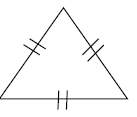• b)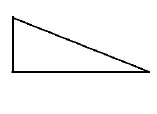• c)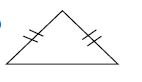#### Question: The centre of the figure is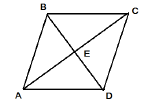• a) E
• b) D
• c) A

#### Question: AB is parallel to CD• a) True
• b) False

#### Question: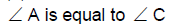• a) True
• b) False

#### Question: AC = BD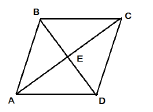• a) False
• b) True

#### Question:• a) False
• b) True

#### Question: E is the midpoint of AC and BD both.• a) True
• b) False

• a)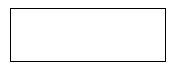• b)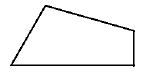• c)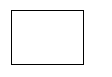• a) circle
• b) hexagon
• c) pentagon

#### Question: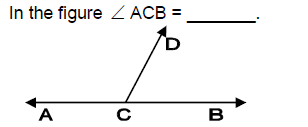• a) 1800
• b) 900
• c) 450

More Questions.....................

#### Question: A triangle can be divided into two equal parts by

• a) angle bisector
• b) circle
• c) line

• a) 900
• b) 150
• c) 300

#### Question: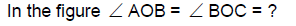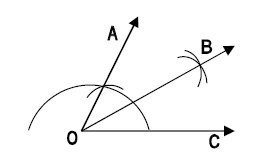• a) 300
• b) 900
• c) 450

#### Question: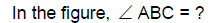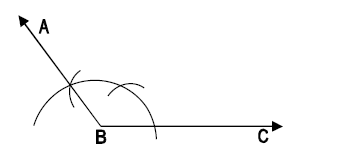• a) 1200
• b) 900
• c) 600

#### Question: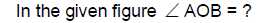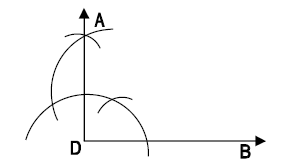• a) 900
• b) 200
• c) 600

#### Question: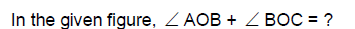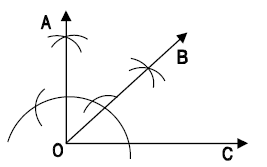• a) 900
• b) 450
• c) 300

• a) line
• b) arc
• c) circle

• a) nonagon
• b) octagon
• c) hexagon

• a) 5
• b) 6
• c) 4

• a) 6
• b) 5
• c) 4

• a) 10
• b) 8
• c) 9

• a) 8
• b) 7
• c) 6

• a) 4
• b) 5
• c) 3

#### Question: Which of the following figure is drawn using a ruler?

• a)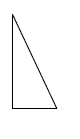• b)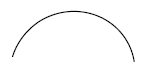• c)